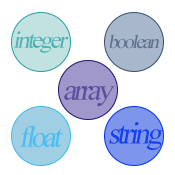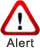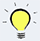# PHP Data TypeWhen you write your name, you use alphabetic characters not numbers. In the same way, when you write your age you use number, not alphabetic characters. So, to represent different information you use different types of data.

## Haw many data types PHP has?

As a language, PHP use some types of data. In general, the data that PHP use can be classified into 8 basic types. These are boolean, integer, float, string, array, object, resource, and NULL.

These 8 primitive types of data are categorized into 3 types

1. Scalar types (Single valued)
• Boolean
• Integer
• Float (floating-point number or double or real number)
• String
2. Compound types (Multi valued)
• Array
• Object
3. Special types
• Resource
• NULL

## Boolean

Boolean data represents the truth. So, it can contain either TRUE or FALSE value.

```<?php
\$var= TRUE;
?>```

In the above example, variable \$var contains TRUE which is a boolean type data.

## Integer

• Any number that is whole number and doesn’t contain fraction in it is integer type data.
• Integer can be negative, positive, or zero. Default sign is positive.
• For example, 10, 1000, -100 are integers but, 10.5 is not an integer as it contains a decimal point.

Integer Base:
Integer type data can be expressed in decimal (base 10), octal (base 8), and hexadecimal (base 16). Most of the time decimal is used.Integer can’t contain thousand separators, commas, blank space etc. It can only contain numbers and positive or negative sign.

Valid range of integer type:

• The minimum and the maximum integer value that PHP supports is platform dependent.
• If it is a 32 bit operating system, then the range is from -2147483648 to 2147483647.
• For 64 bit operating system, the range is from 9223372036854775808 to 9223372036854775807.Integer overflow
If an integer goes beyond its range, PHP converts it from integer type to float type because floating type can store larger value.
```<?php
// In a 32 bit system-
\$number = 2147483647;
var_dump(\$number); //  result: int(2147483647)
\$number = 2147483648;
var_dump(\$number); //  result: float(2147483648)
?>```
var_dump() function displays data type and value of a variable.

PHP stores the maximum value in a constant named PHP_INT_MAX.

```<?php
echo PHP_INT_MAX; // result: 2147483647
?>```

## Floating point number

• Floating point number is also known as float, double, or real number.
• PHP doesn’t support fraction, instead, it expresses fraction in its equivalent floating point number. So, to express 11/4 , 1.25 is written.
• Float can be a positive or a negative number. By default it is a positive number.

How to write floating point number:
Floating point numbers can be written in 2 ways-

1. Using floating point: Ex. 2.45.When you assign 1 PHP treats it as integer type. On the other hand, when you assign 1.0, PHP assigns it as float type
2. Using exponential notation: To express too large or too small numbers, this exponential notation is used. For example. 2.45e3. This type of notation consists of a mantissa, a letter e (or E), and an exponent. In 2.45e3, the mantissa is 2.4 and the exponent is 3. Mantissa could be an integer or a floating point number. Assigning a plus (default) or minus sign in front of it, it defines whether the number is positive or negative. Exponent will always be a whole number and its range from -307 to 308.What does 2.45e3 mean?
2.45e3 can be converted to-
2.45 x 103 = 2.45 x 1000 = 2450Similarly, -2.45e-3 is equivalent to-
-2.45 x 10-3 = -2.45 x .001 = -.00245

Float Base:
Floating point number is always decimal (base 10).

## Null

1. NULL is a special data type. NULL is the only possible value of NULL type of data.
2. NULL value is not equivalent to-
• Mathematical zero(0),
• Empty string, or
• Blank space
```<?php
\$var = 0; // Not NULL
\$var = “”; // Not NULL
\$var = “ ”; Not NULL
?>
```
3. A variable can contain a NULL value if-
• No value is set in it yet.
• Its value set to NULL.
• It value has been unset (using unset(), a variable which has value can be unset.)
```<?php
\$var; //NULL
\$var=NULL; //NULL
\$var=0; //Not NULL
unset(\$var); // NULL
?>
```
• NULL is case insensitive, Null, nULL are all correct.Don’t enclose it in quotes. If it is enclosed in quotes, it will become a string.

```<?php
\$var=NULL; //NULL
\$var=”NULL”; //String
?>```

Other data types – string, array, object, resource will be discussed in other lessons later.

## PHP is loosely typed language

In other word, PHP is a weakly typed or dynamically typed language. When declaring a variable, PHP doesn’t require explicitly which type of data it will contain. You simply assign a value into a variable. In the run time PHP interpreter can recognize the data type of a value that is being stored into a variable. In fact, trying to define a data type explicitly will produce an error. If you try to assign to store a integer into a variable, it will become integer type variable; later, if you assign string into the same variable, it will become string type variable, and so on. This feature is also known as type juggling.

Example-

```<?php
\$var = 7;
\$var = “7”;
\$var = 7.0;
?>```

In the above example, in line 2, as 7 is assigned into \$var variable, its data type is integer; in the next line as “7” is assigned into the same variable, its data type is now string; in the same way, the data type of the value stored in variable \$var in the line 4 is float.

## Type casting

Converting data type from one to another of a value is called type casting. Though PHP interpreter can determines data type of a value itself, but, by type casting data type can be changed.

Syntax

(cast operator) value;

Example:

```<?php
\$var = 10.5;
echo (int)10.5;
\$var2 = (int)\$var;
?>```

In the above example, in line no 2, \$var is a float type variable. In line no 3, using type casting the value 10.5 is being changed to integer 10. So it will print 10. In the line no 4, integer 10 is stored in variable \$var2.

### Casting operators

 Casting operators Convert data type to (bool), (boolean) boolean (int), (integer) integer (float), (double), (real) float (string) string (array) array (object) object (unset) NULL

In the following, we’ll see all the above casting operators in detail

### Converting to Boolean

As boolean only contains TRUE or FALSE value, so converting from other data type to boolean will produce either TRUE or FALSE. The following table shows the converted value for different types of data after boolean type casting.

 FALSE TRUE Boolean FALSE All the remaining values. Integer 0 Float 0.0 Empty string “” or “0” Array with no element NULL SimpleXML objects created from empty tags

Example

```<pre>
<?php
var_dump((bool) FALSE); // return false
var_dump((bool) 0); // return false
var_dump((bool) 0.0); // return false
var_dump((bool) ""); // return false
var_dump((bool) "0"); // return false
var_dump((bool) NULL); // return false
var_dump((bool) array()); // return false
var_dump((bool) ""); // return false
?>
</pre>
```

### Converting to integer

The following shows the converted value for different types of data after integer type casting.

From Boolean
Boolean TRUE will produce 1, and FALSE 0

From float

1. If the floating number is within the range of integer (For a 32 bit system, the range is from -2147483648 to 2147483647 and For 64 bit system, the range is from 9223372036854775808 to 9223372036854775807), then the integer value will be the part of the floating number without the decimal point. Ex, 10.5 will become 10.
2. If the floating point number is outside the range of the integer, then the result will be undefined.

From string

1. If the string starts with numeric data and the numeric value is within the integer range then the converted value will be that numeric value. If it is outside the integer range, then the maximum/minimum integer number will be returned.
2. If the string doesn’t starts with number, then it will return 0.

From other types
The result will be undefined.

```<pre>
<?php
var_dump((int) TRUE); // return 1
var_dump((int) FALSE); // return 0
var_dump((int) -29.90);  // return -29
var_dump((int) 2147483647); // return 2147483647
var_dump((int) "2147483647SchoolsOfWeb"); // return 2147483647
?>
</pre>
```

### Converting to float

The following shows the converted value for different types of data after float type casting.

From Boolean
Boolean TRUE will produce 1, and FALSE 0.

From integer
The value will be as it is.

From string

1. If the string starts with numeric data then the converted value will be that numeric value.
2. If the string doesn’t starts with number, then it will return 0.

From other types
The result will be undefined.

Example

```<pre>
<?php
var_dump((float) TRUE); // return 1
var_dump((float) FALSE); // return 0
var_dump((float) -29); // return -29
var_dump((float) 2147483648); // return 2147483648
var_dump((float) "2147483647SchoolsOfWeb"); // return 2147483647
var_dump((float) "SchoolsOfWeb100"); // return 0
?>
</pre>
```

### Converting to string

Converting a value to string can be done using (string) cast or using the function strval().

From Boolean
Boolean TRUE will produce “1”, and FALSE empty string “”.

From integer/float
The value will be as it is inside the quotation mark. For example 100 will become “100”

From NULL
NULL will produce an empty string “”.

From array, object, or resource
Converting from the array, object, or resource to string will not produce any useful information.

Example:

```<pre>
<?php
var_dump((string) TRUE);  // return “1”
var_dump((string) FALSE); // return “”
var_dump((string) 2.45e3); // return 2450
var_dump((string) 2147483648); // return 2147483648
var_dump((string) NULL); // return “”
?>
</pre>
```

### Converting to array

Converting from integer, float, string, boolean, resource type to array will produce an array which has a single element with index zero. Object converted to array will produce an array with elements of object’s properties.

Example:

```<?php
var_dump((array) 100);
?>```

Result:
array(1){
=>int(100)
}

### Converting to NULL

Casting a value to NULL using (unset) will return NULL

```<pre>
<?php
var_dump((unset)TRUE); // return NULL
var_dump((unset)FALSE); // return NULL
var_dump((unset) -29); // return NULL
var_dump((unset) 2147483648); // return NULL
var_dump((unset)"2147483647SchoolsOfWeb"); // return NULL
var_dump((unset)"SchoolsOfWeb100");  // return NULL
var_dump((unset)"@100"); // return NULL
?>
</pre>
```
 ‹‹ PHP Variables : Previous Lesson Next Lesson: PHP Constants ››